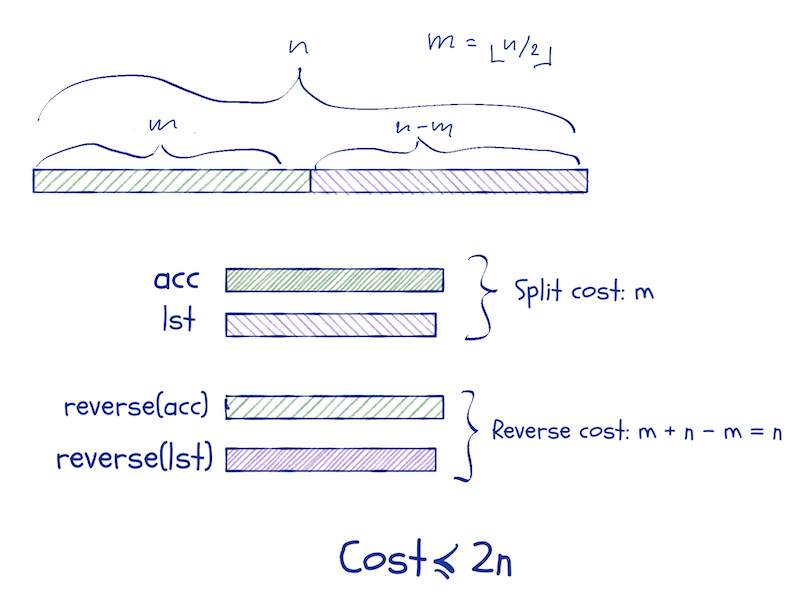# Mailund on the Internet

On Writing, Science, Programming and more

# Errata: Functional Data-Structures in R

One big regret I have about this book is that I didn’t implement the pattern matching code from Domain-Specific Languages in R before this book. Pattern matching makes implementing data structures much easier.

In the errata below, I will use it. You can read a description of how it works here.

To use it, install the patch package and load it

install.packages("pmatch")
library(pmatch)


## Chapter 4

### Deques

This section is just, well, wrong. I made a mistake in the implementation, but it was cancelled out by another error, so it didn’t affect the correctness—so my tests didn’t catch it. It did break the runtime guarantees, though, and my explanation of why that happens is also incorrect.

A correct implementation of deques (to the best of my knowledge), is this:

queue := QUEUE(front_len, front, back_len, back)
new_queue <- function() QUEUE(0, NIL, 0, NIL)

enqueue <- function(queue, x)
cases(queue,
QUEUE(front_len, front, back_len, back) ->
QUEUE(front_len, front, back_len + 1, CONS(x, back)))

enqueue_front <- function(queue, x)
cases(queue,
QUEUE(front_len, front, back_len, back) ->
QUEUE(front_len + 1, CONS(x, front), back_len, back))

split_list <- function(lst, n, acc = NIL)
cases(..(n, lst),
..(0, lst) -> PAIR(reverse(acc), lst),
..(n, CONS(car, cdr)) -> split_list(cdr, n - 1, CONS(car, acc)))

move_front_to_back <- function(queue)
cases(queue,
QUEUE(front_len, front, 0, NIL) -> {
n <- floor(front_len / 2)
cases(split_list(front, n),
PAIR(first, second) ->
QUEUE(n,
first,
front_len - n,
reverse(second)))
})

move_back_to_front <- function(queue)
cases(queue,
QUEUE(0, NIL, back_len, back) -> {
n <- floor(back_len / 2)
cases(split_list(back, n),
PAIR(first, second) ->
QUEUE(back_len - n, reverse(second), n, first))
})

front <- function(queue)
cases(queue,
QUEUE(., NIL, ., NIL)        -> stop("Empty queue"),
QUEUE(., NIL, ., .)          -> front(move_back_to_front(queue)),
QUEUE(front_len, CONS(car, cdr), back_len, back) ->
PAIR(car, QUEUE(front_len - 1, cdr, back_len, back)))

back <- function(queue)
cases(queue,
QUEUE(., NIL, ., NIL)        -> stop("Empty queue"),
QUEUE(., ., ., NIL)          -> back(move_front_to_back(queue)),
QUEUE(front_len, front, back_len, CONS(car, cdr)) ->
PAIR(car, QUEUE(front_len, front, back_len - 1, cdr)))


The cost of splitting a list and moving a list is bounded by 2nso put two operations in the bank each time you increase the difference in list lengths and you can pay for it when you need to.